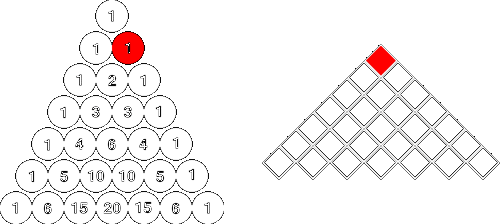# Terms want Freedom

Algebra Level 5Given $p$ and $q$ are positive real numbers that satisfy the equation $p^4 + q^3 = 2$. What is the maximum value of the term independent of $x$ in the expansion below?

$\large \left ( p \space x^{\frac {1}{12}} + q \space x^{-\frac {1}{9}} \right )^{14}$

×